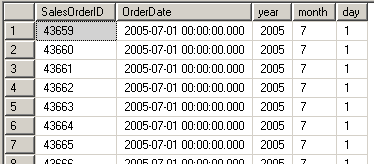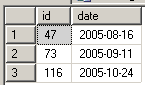﻿ SQL Server Table-Valued Function ExampleSQL Server and T-SQL Development Tutorials Development resources, articles, tutorials, code samples, tools and downloads for ASP.Net, SQL Server, Reporting Services, T-SQL, Windows, AWS, SAP HANA and ABAP

# SQL Server Table-Valued Function Example

A SQL Server table-valued function is a user-defined function which returns a table data type as output.
What makes SQL Server table-valued functions more useful in some situations than sql views or sql stored procedures is like every sql function, a t-sql table-valued function also accepts parameters.
Also TVF's (table valued functions) can be referenced in sql SELECT queries just like a SQL Server database table.
These two features plus creating complex t-sql programming logic in user defined functions make a table-valued function powerful than the SQL view and SQL stored procedure objects.

The most well-known use of SQL Table-Valued Function is sql string split function.
Perhaps sql developers can think of the above example as part of this t-sql tutorial.

The following t-sql table-valued function example can be executed on Microsoft SQL Server 2008 R2 sample database AdventureWorks.

In fact, the above date parser function only returns a single row.
You might prefer to extract Year, Month, and Day date parts within SELECT statements without using a table-valued function.
But please think of udf_ParseDate table-valued function as a starting point in this t-sql tutorial.

The output of the above sql TVF is as follows.In this t-sql tutorial, I want to give a derived date table example too.
The following t-sql table valued function can be used by t-sql programmers in order to create a table where the start and end date ranges are given as a parameter.

T-SQL programmers can use the above user defined table-valued function to find the missing date gaps in SalesOrderHeader table according to the OrderDate column.
Again for this table-valued function example (TVF), I want to use AdventureWorks table.

The following t-sql query which is using TVF - Table Valued Function udf_CreateDateTable will display the dates where no sales order has been created.
Here is the 3 days where no order was created in our AdventureWorks sample database table SalesOrderHeader.SQL Server